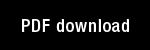On the global limits and oscillations of a system of nonlinear differential equations describing a flow of a probabilistic network

Author(s): Grossberg, S. |

Year: 1969

Citation: Journal of Differential Equations, 5, 531-563

Abstract: 1. INTRODUCTION: This paper considers various aspects of the global limiting and oscillatorybehavior of the following system of nonlinear differential equations.sx;(t) = --Xi(t) +R Z xk(t)Yki(t) +I1(t), (1)kn1YA(t) = zlk(t) [ zfm(t)]-1, (2)M-1- 1k(t) = -uZJk(t) t A(t) xk(t), j k,andz,2(t) = 0, (4)where i,j, k = 1, 2, 3 and is a positiv, number.The system (*) arises as a special case of a nonstationary prediction theoryor learning theory, whose goal is to di;cuss the prediction of individualevents, in a fixed order, and at prescribed tunes (, , ). In this theory,(*) describes a machine .1/ subjected to inputs I = (11 , I2 , I3) by an experimenter f,Â° who records the outputs X - (x1 , x2 , x3) created thereby.' has only the inputs I and the outputs X at his disposal with which todescribe (*), and in terms of these variables (*) takes the formX(t) = -Â«X(t) + B(Xt) X(t) + I(t),

Topics: Mathematical Foundations of Neural Networks, Models: Other,